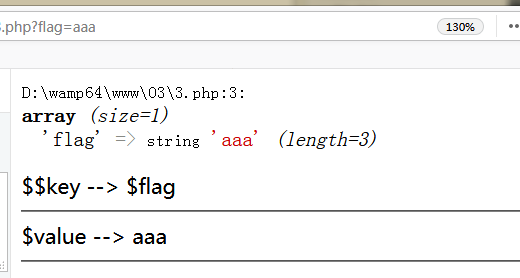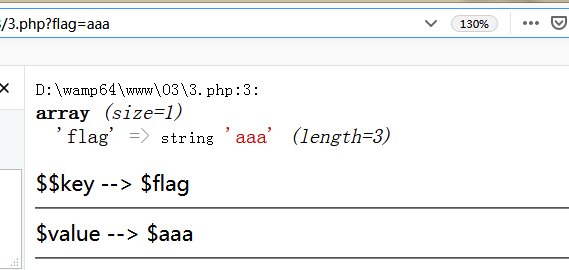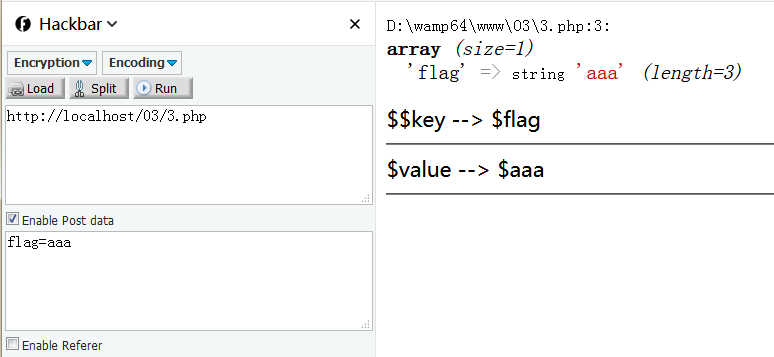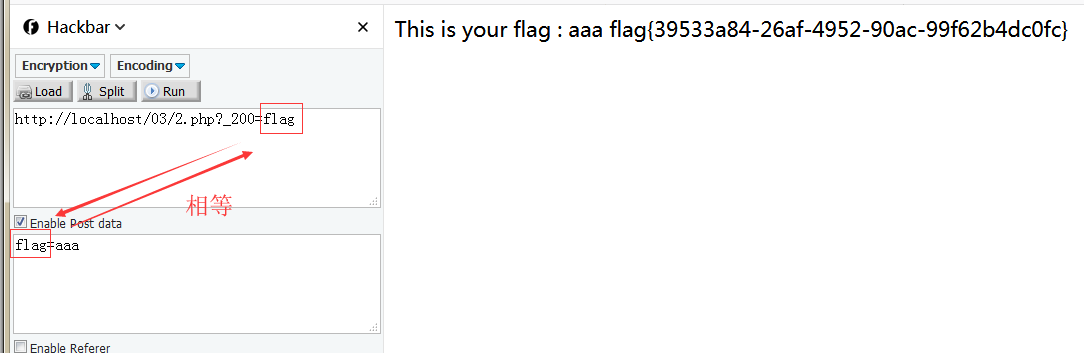相关函数

\$\$extractparse_strimport_request_variables 等等

register_globals 的意思就是注册为全局变量，

1. \$\$可变变量

\$\$ 这种写法称为可变变量，

<?php

\$a = "hello";
echo "\$a".'<hr>';   // 输出 hello
\$a = "world";
echo "\$a".'<hr>';   // 输出 world
echo "\$\$a".'<hr>';  // 输出 \$world

?>

<?php

foreach (\$_GET as \$key => \$value) {
\$\$key = \$value;
echo \$\$key.'<hr>';
}

?><?php
include "flag.php";   // 包含并运行指定文件

if (\$_SERVER["REQUEST_METHOD"] != "POST")   // 返回post的值
{
die("BugsBunnyCTF is here :p...");
}
if ( !isset(\$_POST["flag"]) )   // 检查是否设置，没有设置直接pass
{
die(\$_403);
}
foreach (\$_GET as \$key => \$value)  // 遍历 GET数据
{
\$\$key = \$\$value;
}
foreach (\$_POST as \$key => \$value)  // 遍历 POST数据
{
\$\$key = \$value;
}
if ( \$_POST["flag"] !== \$flag )
{
die(\$_403);
}
echo "This is your flag : ". \$flag . "\n";
die(\$_200);
?>

die() 函数输出一条消息，并退出当前脚本。

foreach (\$_GET as \$key => \$value){  // 遍历 POST数据
\$\$key = \$\$value;
}

$key =$value 的意思是 把键和值分别当作变量名来使用，foreach (\$_POST as \$key => \$value){  // 遍历 GET数据
\$\$key = \$value;
}2. extract()函数

extract() 函数从数组中将变量导入到当前的符号表。

extract_rules 可能值：

1. EXTR_OVERWRITE -     默认。如果有冲突，则覆盖已有的变量。
2. EXTR_SKIP -     如果有冲突，不覆盖已有的变量。
3. EXTR_PREFIX_SAME -     如果有冲突，在变量名前加上前缀 prefix。
4. EXTR_PREFIX_ALL -     给所有变量名加上前缀 prefix。
5. EXTR_PREFIX_INVALID -     仅在不合法或数字变量名前加上前缀 prefix。
6. EXTR_IF_EXISTS -     仅在当前符号表中已有同名变量时，覆盖它们的值。其它的都不处理。
7. EXTR_PREFIX_IF_EXISTS -    仅在当前符号表中已有同名变量时，建立附加了前缀的变量名，其它的都不处理。
8. EXTR_REFS -     将变量作为引用提取。导入的变量仍然引用了数组参数的值。

<?php

\$a = 1;    // 初始值为1
\$b = array('a' => '2');
extract(\$b);    // 经过extract()函数对\$b处理后
echo \$a;    //输出结果为2

?>

<?php if (\$_SERVER["REQUEST_METHOD"] == "POST") { ?>
<?php
extract(\$_POST);
if (\$pass == \$thepassword_123) { ?>
<code><?php echo \$theflag; ?></code>
</div>
<?php } ?>
<?php }
?>

3. parse_str()函数

parse_str() 函数把查询字符串解析到变量中，如果没有array 参数，则由该函数设置的变量将覆盖已存在的同名变量。

1. string   必需。规定要解析的字符串。
2. array   可选。规定存储变量的数组的名称。该参数指示变量将被存储到数组中。

<?php

\$b = 1;
parse_str('b=2');
echo \$b;

?>

<?php
error_reporting(0);
if(empty(\$_GET['id'])) {     // empty()检查是否为空
show_source(__FILE__);     // highlight_file 代码高亮
die();                     // 等同于exit 输出一个消息并且退出当前脚本
} else {
include ('flag.php');
\$a = "www.OPENCTF.com";
\$id = \$_GET['id'];
@parse_str(\$id);
if (\$a != 'QNKCDZO' && md5(\$a) == md5('NKCDZO')) {
echo \$flag;
} else {
exit('其实很简单其实并不难！');
}
}

?>

\$a 设置初始值，用变量\$id来接收GET的数据 ，

https://www.freebuf.com/news/67007.html

?id=a=s1836677006a

?id=a=240610708

s1836677006a md5加密(32位)，也是以 0e 开头，

4. import_request_variables()函数

register_globals被禁止的时候使用

<?php

\$b = 1;
import_request_variables('GP');
echo \$b;

?>

\$b 的值 1 会被覆盖为 2

参考文献

https://www.jianshu.com/p/a4d782e91852

https://www.freebuf.com/column/150731.html